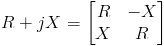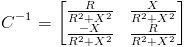# Complex Number Matrix Representation

Published on
11,415 Points
5,215 Views
2 Endorsements
Last Modified:
Approved
Complex Numbers are funny things.  Many people have a basic understanding of them, some a more advanced.  The confusion usually arises when that pesky i (or j for Electrical Engineers) appears and understanding the meaning of a square root of a negative number.  Without having to understand all this, there is a way to do complex algebra (to an extent) without worrying about the i part of the equation.Many people have never used complex numbers in a matrix representation before.  This method allows for a different set of algebra possibilities.  For instance, if you want to divide a complex number by another complex number without going through the rigors of setting up the equation, simply multiply the inverse of the denominator complex times the numerator.  This is much simpler (for large sets of equations) than doing it by hand.

But where does this really help?  Software.

It's much easier for a computer to deal with matrices than complex numbers.  Complex numbers require a separate class and defining all operators.  If you make complex numbers a matrix of real numbers, this process becomes ten times simpler to implement.

Granted there are some advantages to using complex numbers as a separate class.  Admittedly, performing certain operations on a matrix is difficult, like raising e to the matrix M power (in our case, a complex matrix).  Although, this is possible by the Taylor series, it's not as straightforward when doing it numerically.

The four basic operators that are used most often are multiplication, division, subtraction, and addition.  All these can be done with matrix algebra.  The most difficult, of course, is the inverse.  For the sake of those that don't know how, I have also included a matrix showing the inverse of a complex number matrix.After knowing how to calculate the inverse, you can add, subtract, divide, and multiple any complex number in software without having to write a separate class.  Handy right?!

I hope this short article can help in future complex calculatory needs!

Justin
2
Ask questions about what you read
If you have a question about something within an article, you can receive help directly from the article author. Experts Exchange article authors are available to answer questions and further the discussion.
Get 7 days free